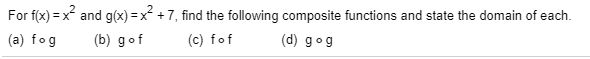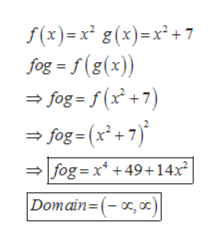# For f(x) = x and g(x) =x +7, find the following composite functions and state the domain of each.%3!(c) fof(b) gof(d) gog(a) fog

Question
6 viewshelp_outlineImage TranscriptioncloseFor f(x) = x and g(x) =x +7, find the following composite functions and state the domain of each. %3! (c) fof (b) gof (d) gog (a) fog fullscreen
check_circle

Step 1

Find the  composite functions and state the domain of each

F(x)= x2

G(x)= x2+7

1. Fog
2. Gof
3. Fof
4. Gog
Step 2

Fog for the functionhelp_outlineImage Transcriptionclosef(x)=x² g(x)=x² + 7 fog = f (g(x)) = fog = f (x +7) = fog = (x² +7) fog =x* +49+14x² Domain=(-x,oc) fullscreen
Step 3

Gof for the fu...

### Want to see the full answer?

See Solution

#### Want to see this answer and more?

Solutions are written by subject experts who are available 24/7. Questions are typically answered within 1 hour.*

See Solution
*Response times may vary by subject and question.
Tagged in

### Other